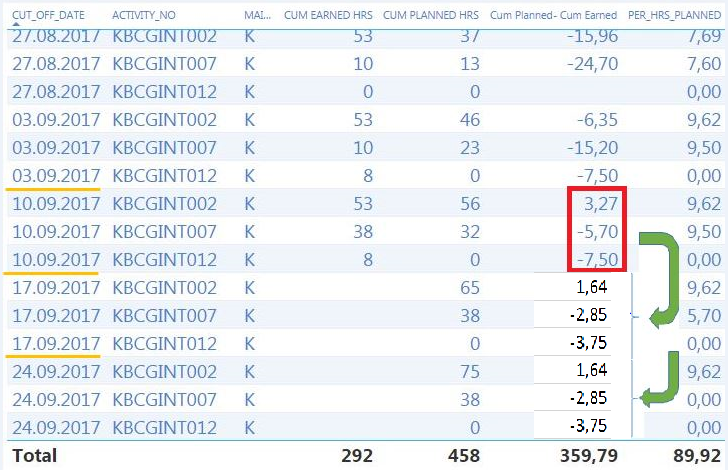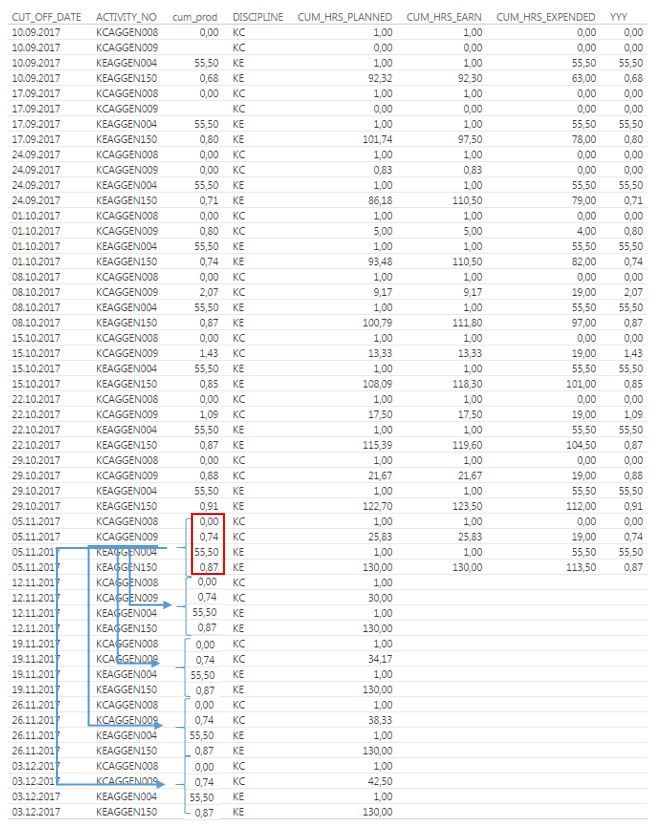cancel
Showing results for
Did you mean:

Fabric is Generally Available. Browse Fabric Presentations. Work towards your Fabric certification with the Cloud Skills Challenge.Resolver I

## DAX Power BI Desktop

Hi,

I am trying to show the difference between Panned Hrs Earned Hrsided by 2 (2= 2 next weeks) per activity based on last cut off date and add this data to the next 2 weeks (e.g. 2 next cut off dates) to the corresponding activity no.

The below example is illustrated in the table below, which also shows the calculations. If anyone could help me out that would be great 🙂.

Rgds

Henrik

2 ACCEPTED SOLUTIONSMicrosoft

@Henrik_99

In this scenario, you need to get the last non blank Panned-Earned's Cut_Off_Date, have it compare with current date to determine if it should return half of the last non blank Panned-Earned. Please refer to my sample below:

```LastNonBlankDate =
CALCULATE (
MAX ( 'Table'[Cut_Off_Date] ),
FILTER (
'Table',
'Table'[Cut_Off_Date] < EARLIER ( 'Table'[Cut_Off_Date] )
&& 'Table'[ID] = EARLIER ( 'Table'[ID] )
&& 'Table'[Panned-Earned] <> BLANK ()
)
)```
`DateDiff = 1*('Table'[Cut_Off_Date]-'Table'[LastNonBlankDate])`
```Column =
IF (
'Table'[Panned-Earned] = 0,
IF (
'Table'[DateDiff] <= 14,
CALCULATE (
LASTNONBLANK ( 'Table'[Panned-Earned], 1 ),
FILTER (
'Table',
'Table'[Cut_Off_Date] < EARLIER ( 'Table'[Cut_Off_Date] )
&& 'Table'[ID] = EARLIER ( 'Table'[ID] )
)
)
/ 2,
BLANK ()
),
'Table'[Panned-Earned]
)
```

If you just want to build one column, it can be like:

```Column =
IF (
'Table'[Panned-Earned] = 0,
IF (
1
* (
'Table'[Cut_Off_Date]
- CALCULATE (
MAX ( 'Table'[Cut_Off_Date] ),
FILTER (
'Table',
'Table'[Cut_Off_Date] < EARLIER ( 'Table'[Cut_Off_Date] )
&& 'Table'[ID] = EARLIER ( 'Table'[ID] )
&& 'Table'[Panned-Earned] <> BLANK ()
)
)
)
<= 14,
CALCULATE (
LASTNONBLANK ( 'Table'[Panned-Earned], 1 ),
FILTER (
'Table',
'Table'[Cut_Off_Date] < EARLIER ( 'Table'[Cut_Off_Date] )
&& 'Table'[ID] = EARLIER ( 'Table'[ID] )
)
)
/ 2,
BLANK ()
),
'Table'[Panned-Earned]
)
```Regards,Resolver I

Hi Simon,

Thank you very much for your reply. I need to verify the solution in my desktop and give you a Feedback, thanks.

Regards

Henrik

3 REPLIES 3Resolver I

Hi,

Just additional question. The soultion worked successfully for Calculated colums. What about for measures, see example below?

Thanks.

Rgds

H.Microsoft

@Henrik_99

In this scenario, you need to get the last non blank Panned-Earned's Cut_Off_Date, have it compare with current date to determine if it should return half of the last non blank Panned-Earned. Please refer to my sample below:

```LastNonBlankDate =
CALCULATE (
MAX ( 'Table'[Cut_Off_Date] ),
FILTER (
'Table',
'Table'[Cut_Off_Date] < EARLIER ( 'Table'[Cut_Off_Date] )
&& 'Table'[ID] = EARLIER ( 'Table'[ID] )
&& 'Table'[Panned-Earned] <> BLANK ()
)
)```
`DateDiff = 1*('Table'[Cut_Off_Date]-'Table'[LastNonBlankDate])`
```Column =
IF (
'Table'[Panned-Earned] = 0,
IF (
'Table'[DateDiff] <= 14,
CALCULATE (
LASTNONBLANK ( 'Table'[Panned-Earned], 1 ),
FILTER (
'Table',
'Table'[Cut_Off_Date] < EARLIER ( 'Table'[Cut_Off_Date] )
&& 'Table'[ID] = EARLIER ( 'Table'[ID] )
)
)
/ 2,
BLANK ()
),
'Table'[Panned-Earned]
)
```

If you just want to build one column, it can be like:

```Column =
IF (
'Table'[Panned-Earned] = 0,
IF (
1
* (
'Table'[Cut_Off_Date]
- CALCULATE (
MAX ( 'Table'[Cut_Off_Date] ),
FILTER (
'Table',
'Table'[Cut_Off_Date] < EARLIER ( 'Table'[Cut_Off_Date] )
&& 'Table'[ID] = EARLIER ( 'Table'[ID] )
&& 'Table'[Panned-Earned] <> BLANK ()
)
)
)
<= 14,
CALCULATE (
LASTNONBLANK ( 'Table'[Panned-Earned], 1 ),
FILTER (
'Table',
'Table'[Cut_Off_Date] < EARLIER ( 'Table'[Cut_Off_Date] )
&& 'Table'[ID] = EARLIER ( 'Table'[ID] )
)
)
/ 2,
BLANK ()
),
'Table'[Panned-Earned]
)
```Regards,Resolver I

Hi Simon,

Thank you very much for your reply. I need to verify the solution in my desktop and give you a Feedback, thanks.

Regards

HenrikAnnouncements#### Power BI Monthly Update - November 2023

Check out the November 2023 Power BI update to learn about new features.#### Fabric Community News unified experience

Read the latest Fabric Community announcements, including updates on Power BI, Synapse, Data Factory and Data Activator.#### The largest Power BI and Fabric virtual conference

130+ sessions, 130+ speakers, Product managers, MVPs, and experts. All about Power BI and Fabric. Attend online or watch the recordings.Top Solution Authors
Top Kudoed Authors
Users online (1,816)